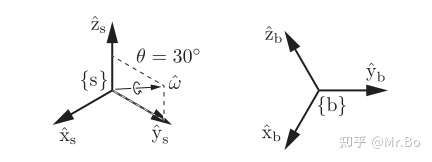The exponential coordinates parametrize a rotation matrix in terms of a rotation axis (represented by a unit vector) and an angle of rotation θ about that axis; the vectorthen serves as the three-parameter exponential coordinate representation of the rotation.

（1）如果定系s绕轴旋转θ,新的位置相对于定系将由旋转矩阵R表达

（2）the angular velocityexpressed in {s} such that, if a frame initially coincident with {s} followedfor one unit of time (i.e.,is integrated over this time interval), its final orientation would be expressed by R.

(3)the angular velocityexpressed in {s} such that, if a frame initially coincident with {s} followedfor θ units of time (i.e., θ is integrated over this time interval) its final orientation would be expressed by R.（1）绕某轴）旋转（2）将（1）中两者相乘形成的三维向量时，引入矩阵的迹：时，,

（1）如果,意味着且旋转轴没有被定义

（2）如果，意味着,旋转轴的表示为下式三者任意一个：

（3）如果,那么：